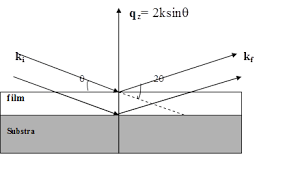## How to Calculate and Solve for Resolution X-rays | X-Ray CrystallographyThe image above represents resolution x-rays.

To compute for resolution x-rays, two essential parameters are needed and these parameters are Average Height of Voltage Pulse (V) and Spread of Voltage Pulse (δv).

The formula for calculating resolution x-rays:

R% = 100 (√(δv)/V)

Where:

R% = Resolution X-rays
V = Average Height of Voltage Pulse
δv = Spread of Voltage Pulse

Let’s solve an example;
Find the resolution x-rays when the average height of voltage pulse is 21 and the spread of voltage pulse is 10.

This implies that;

V = Average Height of Voltage Pulse = 21
δv = Spread of Voltage Pulse = 10

R% = 100 (√(δv)/V)
R% = 100 (√(10)/21)
R% = 100 (3.162/21)
R% = 100 (0.15)
R% = 15.05

Therefore, the resolution x-rays is 15.05%.

Calculating the Average Height of Voltage Pulse when the Resolution X-rays and the Spread of Voltage Pulse is Given.

V = 100 x √(δv) / R%

Where;

V = Average Height of Voltage Pulse
R% = Resolution X-rays
δv = Spread of Voltage Pulse

Let’s solve an example;
Find the average height of voltage pulse when the resolution x-rays is 40 and the spread of voltage pulse is 4.

This implies that;

R% = Resolution X-rays = 40
δv = Spread of Voltage Pulse = 4

V = 100 x √(δv) / R%
V = 100 x √(4) / 40
V = 100 x 2 / 40
V = 200 / 40
V = 5

Therefore, the average height of voltage pulse is 5.